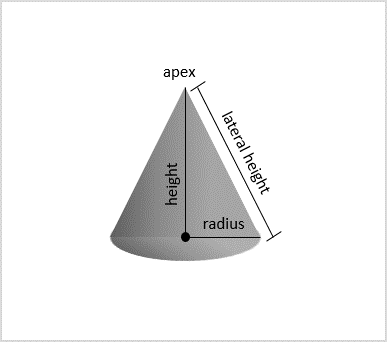# Python Program to calculate the volume and area of Cone

A cone is a three-dimensional figure that is formed by connecting infinite line segments from a common point to all the points in a circular base. This common point is also known as an apex. The cone is measured using three dimensions: radius of its circular base, height and lateral height.

The difference between a cone’s height and lateral height is such that− height is measured from the apex to the center of the circular base, while lateral height is a length of line segments connecting apex and any point on the circular base.The lateral surface area, also known as curved surface area, of a cone is measured using the lateral height and the total surface area is also measured using the lateral height plus the area of circular base. The formulae to calculate these areas is as follows

Lateral Surface Area − πrl
Total Surface Area − πr(r+l)


The volume of a cone is defined as the space contained by the curved surface and circular base of the cone.

Volume − $\mathrm{{\frac{1}{3}\pi r^2h}}$

### Input Output Scenarios

Let us look at some input output scenarios −

Assume the inputs taken as the radius of the circular base, actual height and lateral height, the output is obtained as −

Input: (3, 4, 5) // 3 is radius, 4 is actual height and 5 is lateral height
Result: Lateral Surface Area: 47.12388980384689
Total Surface Area: 75.39822368615503
Volume: 37.69911184307752


## Using Mathematical Formulae

We use standard mathematical formulae to find the surface areas and volume of a cone. The input requirements are radius, lateral height and actual height of the cone. Let us look a simple python example to understand it better.

### Example

Following example implements the calculation of surface areas and volume of a cone with a certain radius and heights.

import math

l = 5
h = 4
r = 3

#calculating the lateral surface area
lsa = (math.pi)*r*l
print("Lateral Surface Area: ", str(lsa))

#calculating the total surface area
tsa = (math.pi)*r*(r+l)
print("Total Surface Area: ", str(tsa))

#calculating the volume
vol = (1/3)*(math.pi)*r*r*h
print("Volume: ", str(vol))


### Output

On compiling and executing the program above, the output is obtained as −

Lateral Surface Area: 47.12388980384689
Total Surface Area: 75.39822368615503
Volume: 37.69911184307752


## Function to Calculate Area and Volume

Python also allows user-defined functions which can be declared using the def keyword with as many arguments required. In this example, we will create functions to calculate the surface areas and volume of the cone.

### Example

In the following example, the input taken by the program will be radius, height and lateral height. User-defined functions are declared to calculate the surface areas and volume.

import math
def cone_lsa(r, l):
#calculating the lateral surface area
lsa = (math.pi)*r*l
print("Lateral Surface Area: ", str(lsa))

def cone_tsa(r, l):
#calculating the total surface area
tsa = (math.pi)*r*(r+l)
print("Total Surface Area: ", str(tsa))

def cone_vol(r, h):
#calculating the volume
vol = (1/3)*(math.pi)*r*r*h
print("Volume: ", str(vol))

l = 5
h = 4
r = 3
cone_lsa(r, l)
cone_tsa(r, l)
cone_vol(r, h)


### Output

The output for the code above is displayed as follows −

Lateral Surface Area: 47.12388980384689
Total Surface Area: 75.39822368615503
Volume: 37.69911184307752